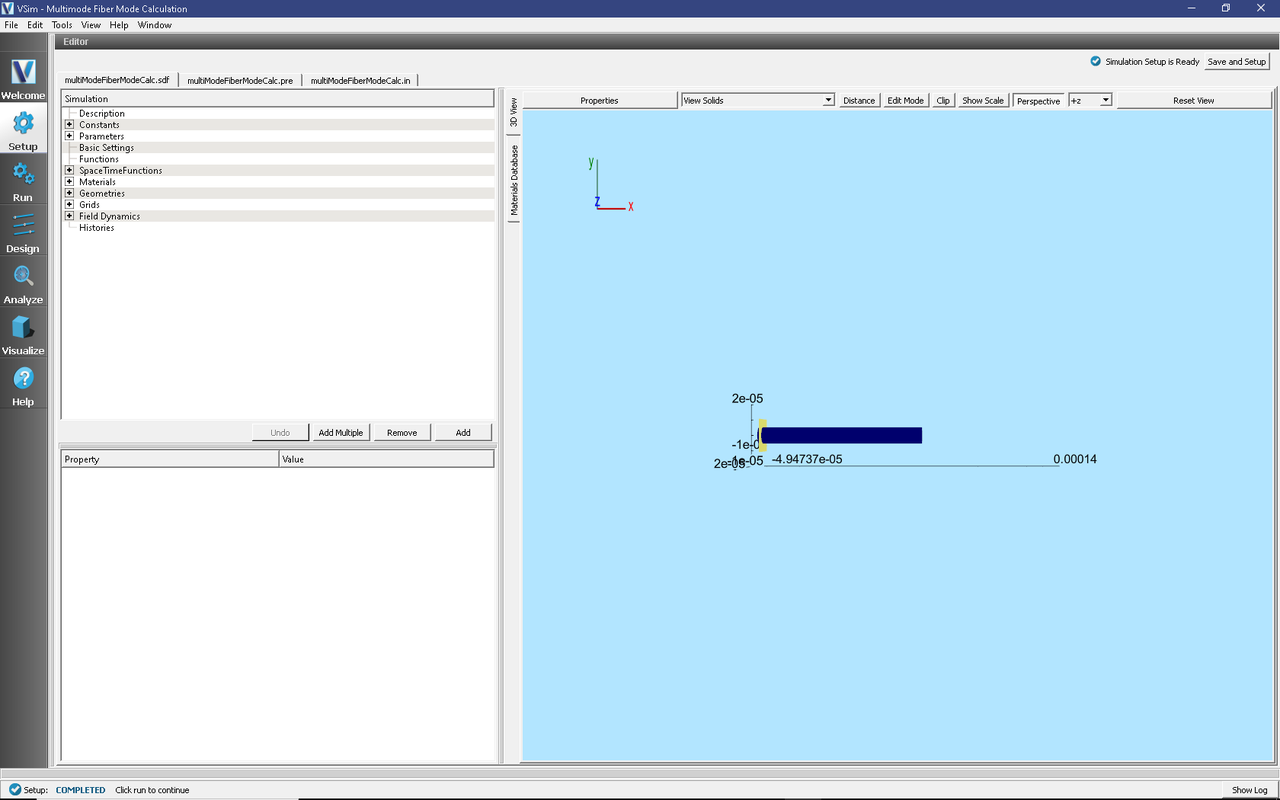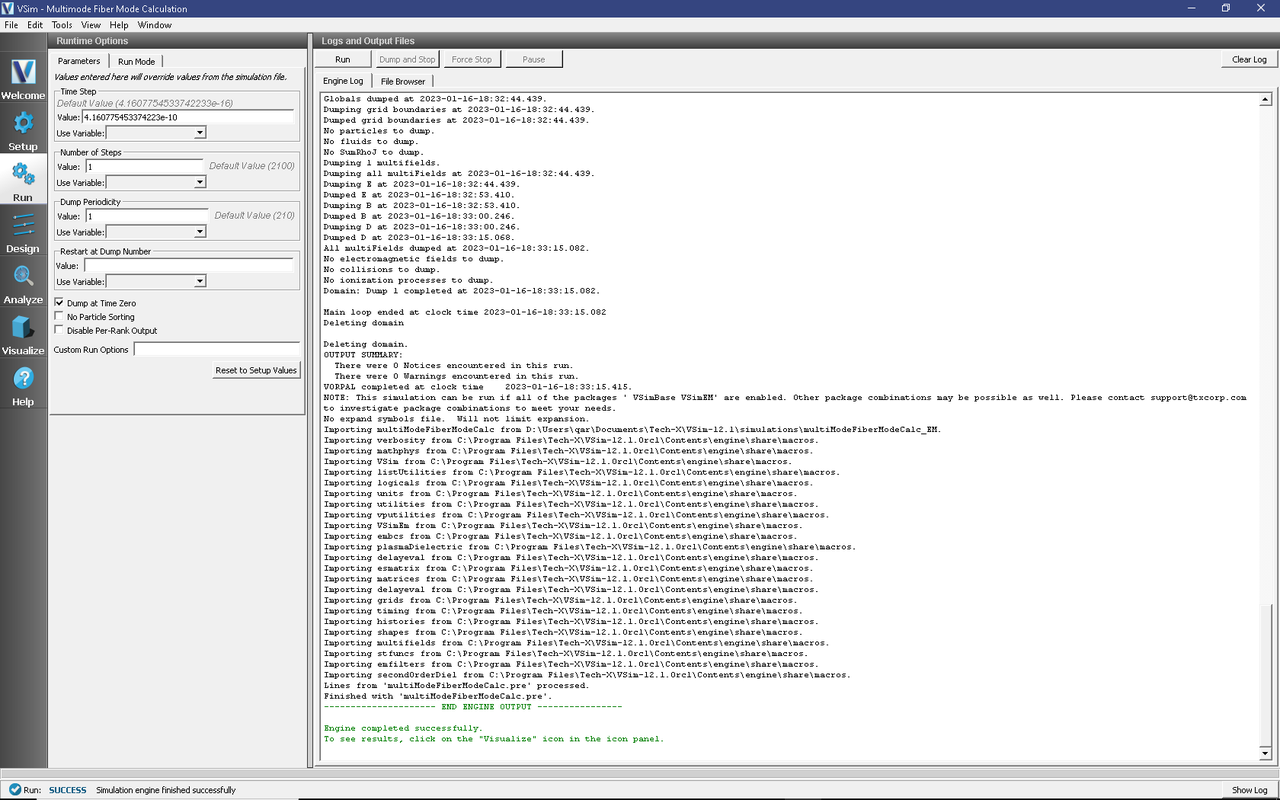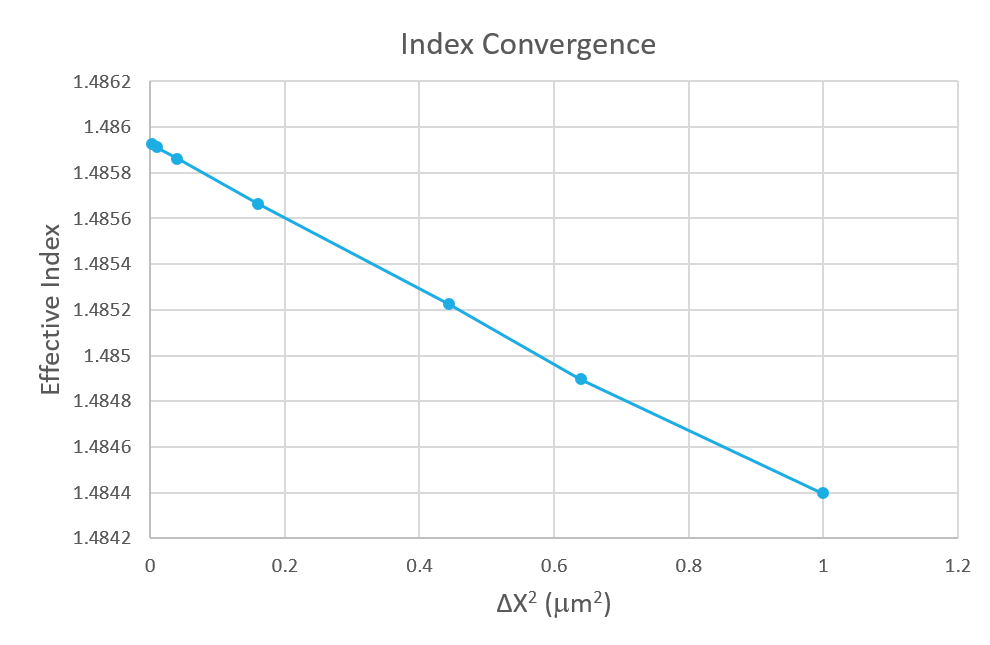# Multimode Fiber Mode Calculation (multiModeFiberModeCalc.sdf)

Keywords:

Mode Extraction, Photonic Waveguide, Guided Mode, Semiconductor

## Problem Description

This example demonstrates the process for extracting the effective index and fields of a guided mode by directly solving an eigenvalue equation. The use of permittivity averaging enables second order accuracy in our solution. The waveguide axis runs parallel to the x-axis, and is surrounded by a background cladding with a greater permittivity. We will run the simulation for 1 step and then use the multiModeFiberModeCalc_invEps_0.h5 file to solve for the guided modes using the computeDielectricModes.py analyzer. This analyzer will find the entire basis set of modes for this fiber and output each into a separate .vsh5 file. These mode files can be used to launch the exact modes into your simulation. This process is shown in the multiModeFiberModeLaunchT example.

Eigenmodes in such a simulation have the form:

$\mathbf{E}(\mathbf{x},t) = \mathbf{E}(y,z) e^{i(k x - \omega t)}$

The effective index of refraction of a waveguide mode is given by $$\bar{n} = k / k_0$$ where $$k_0 = \omega / c$$. If the waveguide has index of refraction $$n_w$$ and the cladding $$n_c < n_w$$, then a guided mode will have a modal index in the range, $$n_c < \bar{n} < n_w$$.

This simulation can be performed with a VSimEM license.

## Opening the Simulation

To open this example open an instance of VSimComposer and follow the steps below:

• In the resulting Examples window expand the VSim for Electromagnetics option.
• Expand the Photonics option.
• Select Multimode Fiber Mode Calculation and press the Choose button.
• In the resulting dialog, create a New Folder if desired, and press the Save button to create a copy of this example.

## Simulation Variables

Constants This example contains a number of constants defined to make the simulation easily modifiable.

• WAVELENGTH_VAC: The vacuum wavelength (frequency divided by the speed of light) for the eigenmode of interest. In this example we scale all lengths by 1e6. Therefore, a typical 1.55 micron source is simply 1.55 in this example.
• NA: The numerical aperture of the fiber. This defines the background permittivity relative to the core permittivity.
• PERMITTIVITY_CORE: This is the permittivity of the core material as defined in the Materials branch and assigned in the Geometries branch.
• RESOLUTION_YZ: The number of cells per core radius in the transverse (y & z) directions.
• RESOLUTION_X: The number of cells per estimated wavelength in the propagation (x) direction.
• CFL_NUMBER: The time step, DT, will be this value times the limit for numerical stability.

Parameters Many parameters in this simulation are defined to assist with launching the mode in a subsequent example, multiModeFiberModeLaunchT. Some important parameters that are relevant to the mode extraction are given below.

• PERMITTIVITY_BG: The permittivity assigned to the background surrounding the core. This is assigned in the Basic Settings branch under background permittivity.
• NMODES: The approximate number of modes supported by the fiber. Depends on NA, RADIUS_CORE, & WAVELENGTH_VAC. Includes degenerate modes. More accurate for many modes (>20).
• BGNYZ_SOURCE: The starting position for where we will evaluate the transverse permittivity profile on the analyzer tab. Defined for convenience and clarity. This slice is shown as the “source” geometry in the 3D view.
• ENDYZ_SOURCE: The ending position for where we will evaluate the transverse permittivity profile on the analyzer tab.

## Setting up the Simulation

As delivered, the system is set up to generate the data needed to run the computeDielectricModes.py analyzer. To ensure that your simulation has second order accuracy, expand the Basic Settings branch and verify that the dielectric solver field is set to permittivity averaging. This algorithm is a powerful VSim feature. This setting is shown in Fig. 227.Fig. 227 Choosing the second order accurate, permittivity averaging for the dielectric solver field under Basic Settings.

## Running the Simulation

After performing the above actions, continue as follows:

• Proceed to the Run Window by pressing the Run button in the left column of buttons. You will be asked to Save. Click Save upon the request to save.
• In the left pane change the Number of Steps and Dump Periodicity to 1.
• Under Additional Run Options select Dump at Time Zero.
• To run the file, click on the Run button in the upper left corner of the right pane. You will see the output of the run in the right pane. The run has completed when you see the output, “Engine completed successfully.” The result is shown in Fig. 228.Fig. 228 Running the simulation for one step to get the permittivity data for the analyzer. Note that Dump at Time Zero is checked.

## Solving for the Eigenmodes

After performing the above actions, continue as follows:

• Proceed to the Analyze Window by clicking the Analyze button on the left.
• Select computeDielectricModes.py and click Open under the list.

Now update the analyzer fields accordingly. Some of these parameters are described above under Parameters

• transverseSlice: 0,-10:10,-10:10
• vacWavelength: 1.55
• nModes: 25
• writeFieldProfile: H,E,D

We set the number of modes (nModes) to a value greater than the number of modes we expect. The analyzer will only find guided modes. Also check Overwrite Existing Files. Run the analyzer by clicking Analyze button in the upper right corner. The analyzer output should resemble Fig. 229. We see that the analyzer found 20 modes. They are listed in decreasing order of effective index. After the modes are calculated, the analyzer will dump the solutions to file so they can be visualized - this may take a few minutes.Fig. 229 The analyzer window after a successful run of computeDielectricModes.py.

## Visualizing the Results

After performing the above actions proceed to the Visualize window by pressing the Visualize button in the left column of buttons. You may need to Reload Data (bottom left). Visualize an eigenmode by following these steps:

• From the Data View dropdown, select Data Overview.
• Expand Scalar Data, expand EigenD, and select EigenD_z.
• At the top of the visualization window, tick the Full Frame box.
• Below the visualization, the dump slider will allow you to scroll between the modes. Scroll to dump number 13.

The resulting visualization pane should resemble Fig. 230.Fig. 230 The visualization pane showing the z component of the D field of the M=1, L=3 mode.

One can select other components of the H, E, or D field to see how they vary for the eigenmodes. These eigenmodes are now saved in .vsh5 files in the folder where the simulation was run.

## Further Experiments

Increase the radius, decrease the wavelength, or increase the numerical aperture on the Setup window and rerun the simulation and analyzer to see higher order modes.

Once you have your desired mode, launch it down the waveguide using the procedure laid out in the multiModeFiberModeLaunchT example.

One can run a full convergence study of eigenmode effective indices by varying the RESOLUTION_YZ constant in the Setup window and re-running the simulation and mode extraction script. A plot of the effective index as a function of transverse cell area is shown in Fig. 231. The linear relationship shows the second order accuracy of our dielectric algorithms.Fig. 231 The effective index as a function of transverse cell area for an eigenmode.